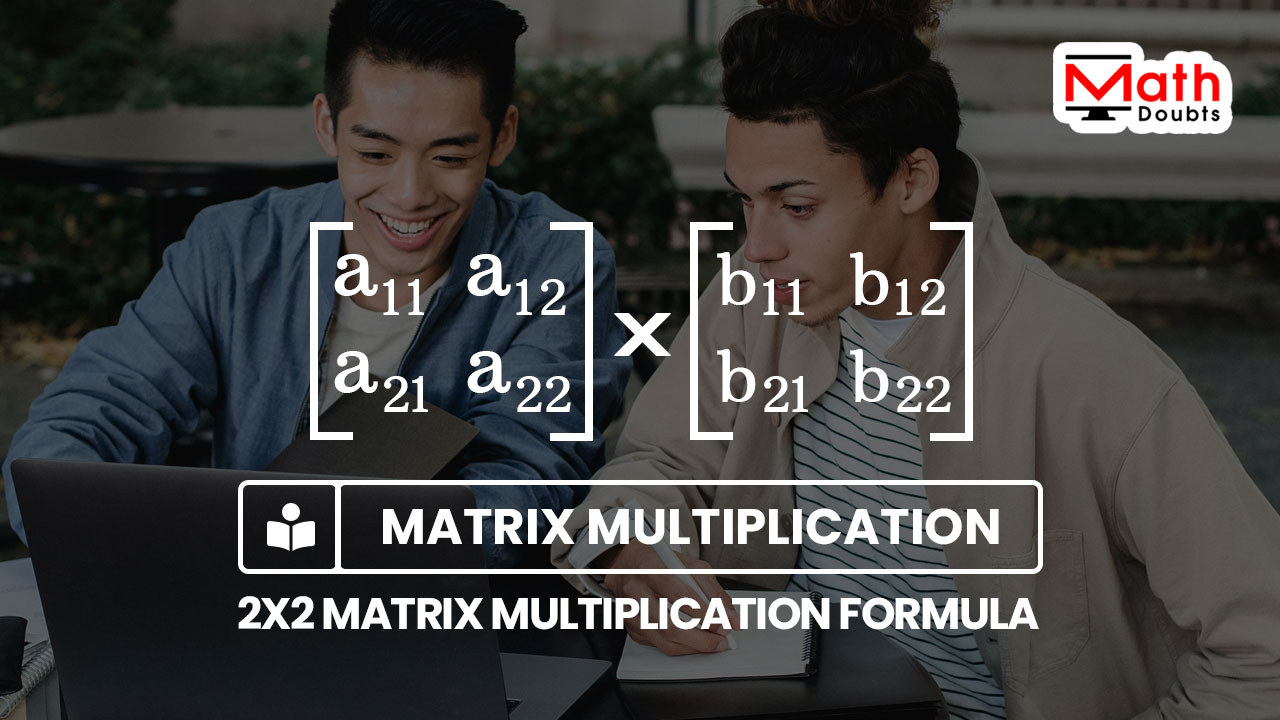# Multiplying the 2×2 Matrices formula

## Formula

${\begin{bmatrix} a_{11} & a_{12} \\ a_{21} & a_{22} \\ \end{bmatrix}}$ $\times$ ${\begin{bmatrix} b_{11} & b_{12} \\ b_{21} & b_{22} \\ \end{bmatrix}}$ $\,=\,$ ${\begin{bmatrix} a_{11}b_{11}+a_{12}b_{21} & a_{11}b_{12}+a_{12}b_{22} \\ a_{21}b_{11}+a_{22}b_{21} & a_{21}b_{12}+a_{22}b_{22} \\ \end{bmatrix}}$

### Introduction

The fundamental process of matrix multiplication takes more time to calculate the product of any two or more $2 \times 2$ matrix. So, it is essential to derive a formula to evaluate the product and it is called the $2 \times 2$ matrix multiplication formula. Now, let’s clearly learn what the multiplication of $2 \times 2$ matrices formula is.Let $a_{11},$ $a_{12},$ $a_{21}$ and $a_{22}$ denote four entries in a square matrix of order $2$. Similarly, $b_{11},$ $b_{12},$ $b_{21}$ and $b_{22}$ represent four elements in another $2 \times 2$ matrix. The multiplication of the $2 \times 2$ square matrix is written mathematically in algebraic form as follows.

${\begin{bmatrix} a_{11} & a_{12} \\ a_{21} & a_{22} \\ \end{bmatrix}}$ $\times$ ${\begin{bmatrix} b_{11} & b_{12} \\ b_{21} & b_{22} \\ \end{bmatrix}}$

The product of the two $2$ by $2$ matrix can be obtained by using the procedure of multiplying the matrices, and the product of them can be written in matrix form as follows.

$\,=\,$ ${\begin{bmatrix} a_{11}b_{11}+a_{12}b_{21} & a_{11}b_{12}+a_{12}b_{22} \\ a_{21}b_{11}+a_{22}b_{21} & a_{21}b_{12}+a_{22}b_{22} \\ \end{bmatrix}}$

It is used as a formula directly to calculate the product of two or more two by two square matrix.

#### Example

Evaluate ${\begin{bmatrix} 1 & 2 \\ 3 & 4 \\ \end{bmatrix}}$ $\times$ ${\begin{bmatrix} 8 & 7 \\ 6 & 5 \\ \end{bmatrix}}$

Let’s take that ${\begin{bmatrix} a_{11} & a_{12} \\ a_{21} & a_{22} \\ \end{bmatrix}}$ $\,=\,$ ${\begin{bmatrix} 1 & 2 \\ 3 & 4 \\ \end{bmatrix}}$ and ${\begin{bmatrix} b_{11} & b_{12} \\ b_{21} & b_{22} \\ \end{bmatrix}}$ $\,=\,$ ${\begin{bmatrix} 8 & 7 \\ 6 & 5 \\ \end{bmatrix}}$

The comparison of the two matrices of order $2$ with the formula of $2 \times 2$ matrix multiplication clears that $a_{11}$ $\,=\,$ $1$, $a_{12}$ $\,=\,$ $2$, $a_{21}$ $\,=\,$ $3$, $a_{22}$ $\,=\,$ $4$, $b_{11}$ $\,=\,$ $8$, $b_{12}$ $\,=\,$ $7$, $b_{21}$ $\,=\,$ $6$ and $b_{22}$ $\,=\,$ $5$.

Now, substitute the values in the multiplication of $2 \times 2$ matrices formula in algebraic form to find the product of the given two matrices in this example.

$\implies$ ${\begin{bmatrix} a_{11}b_{11}+a_{12}b_{21} & a_{11}b_{12}+a_{12}b_{22} \\ a_{21}b_{11}+a_{22}b_{21} & a_{21}b_{12}+a_{22}b_{22} \\ \end{bmatrix}}$ $\,=\,$ ${\begin{bmatrix} 1 \times 8+2 \times 6 & 1 \times 7+2 \times 5 \\ 3 \times 8+4 \times 6 & 3 \times 7+4 \times 5 \\ \end{bmatrix}}$

$\,\,=\,$ ${\begin{bmatrix} 8+12 & 7+10 \\ 24+24 & 21+20 \\ \end{bmatrix}}$

$\,\,=\,$ ${\begin{bmatrix} 20 & 17 \\ 48 & 41 \\ \end{bmatrix}}$

#### Proof

Learn how to derive the multiplication of $2$ by $2$ matrices formula in algebraic form by the procedure of finding product of two square matrix of order $2$.

Latest Math Topics
Jun 26, 2023
Jun 23, 2023

Latest Math Problems
Jul 01, 2023
Jun 25, 2023
###### Math Questions

The math problems with solutions to learn how to solve a problem.

Learn solutions

Practice now

###### Math Videos

The math videos tutorials with visual graphics to learn every concept.

Watch now

###### Subscribe us

Get the latest math updates from the Math Doubts by subscribing us.